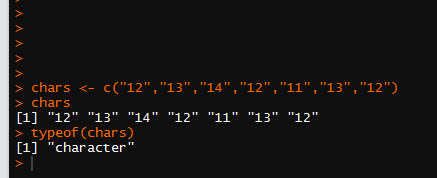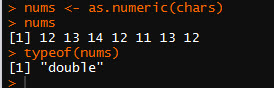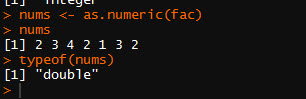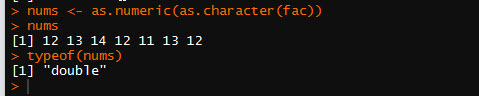# R: Converting Factors to Numbers

R, like all programming languages, has its quirks. One of the more frustrating ones is the way it acts when trying to convert a factor into a numeric variable.

```chars <- c("12","13","14","12","11","13","12")
chars
typeof(chars)```

Here is the outputNow, let’s convert this vector to a numeric vector using the function as.numeric()

```nums <- as.numeric(chars)
nums
typeof(nums)```

And here is the outputAs you can see it works fine.

But now let’s try it with a factor

```fac <- factor(c("12","13","14","12","11","13","12"))
fac
typeof(fac)```

Here is the output.Now look what happens when I try the as.numeric() function

```nums <- as.numeric(fac)
nums
typeof(nums)```

Check out the resultsWhile is says the type is a double, clearly the numbers are not correct.

## How to fix it?

Well, the secret is that first you need to convert the factor into a character, then into a numeric.

```nums <- as.numeric(as.character(fac))
nums
typeof(nums)```

Now check out the resultsNow we have the correct numbers. Just keep this little trick in mind. It has caused me some undue frustration in past.